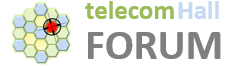Hello,
Im bit new to phy concepts.
Actually googling gave me:
### RSSI = wideband power = noise + serving cell power + interference power

So, without noise and interference, we have that 100% DL PRB activity: RSSI=12 * N * RSRP

But my doubt is : Since RSRP is power of SINGLE RE. RSSI should correspond to power of single RE taking noise and interference into account. But then above formula should have been
RSSI = 12 * N * RSRP * 7

Because each RB in LTE is 7 symbols and 12 subcarriers ?

why is the 7 missing in the above equation

thanks a lot

RSRP is the average power of RE carrying Cell Specific Reference signal while the RSSI is the total Wideband Power Measured across the symbol Carrying Cell Specific Reference signal. Cell specific Reference signal exist for one symbol at a time.

RSSI measured only in symbol carrying RS Symbol in RB- so if cell is not carrying any traffic than only RS will be transmitted. So lets;s say in First symbol if nothing is transmitted than symbol will carry 2 RS only.
Lets say= RSRP= 1watt
So for cell with No Load:( 1 RB)

For Full load condition where RE are carrying Traffic and CRS
RSSI=N * 12 RSRP =1121=12 watt

RSSI and RSRP is measured on same OFDM Symbol carrying RS–Not for all the OFDM Symbols…that is the reason 3GPP Formula for RSSI is 12NRSRP not RSSI=12NRSRP*7

I hope it clears your doubt.

Thanks
Govind Dahiya

Thanks
Govind Dahiya

1 Like

Excellent, thankyou very much

Thanks for sharing